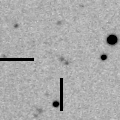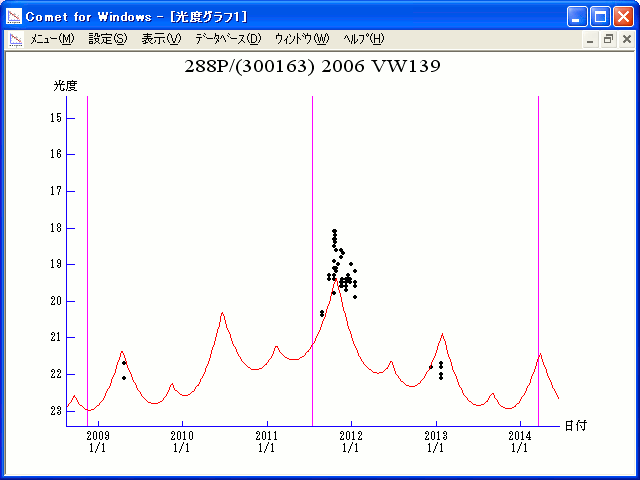# 2006 VW139\$BWB@1(B

288P/(300163) 2006 VW139 (2011)###\$B###\$B50F;MWAG(B

```                    Epoch = 2011 Aug. 27.0 TT
T = 2011 July 18.5417 TT         Peri. = 281.8862
e = 0.201125                     Node  =  83.2065  2000.0
q = 2.437865 AU                  Incl. =   3.2392
a =  3.051625 AU    n = 0.1848874    P =   5.33 years
```

###\$B8wEYJQ2=(B

```        H = 16.3  G = 0.15
```##### \$B50F;MWAG\$O!"(BCBET 2920\$B\$K7G:\\$5\$l\$?\$b\$N\$G\$9!#(B \$B8wEY%0%i%U\$O(BComet for Windows\$B\$G:n@.\$7\$?\$b\$N\$G\$9!#(B